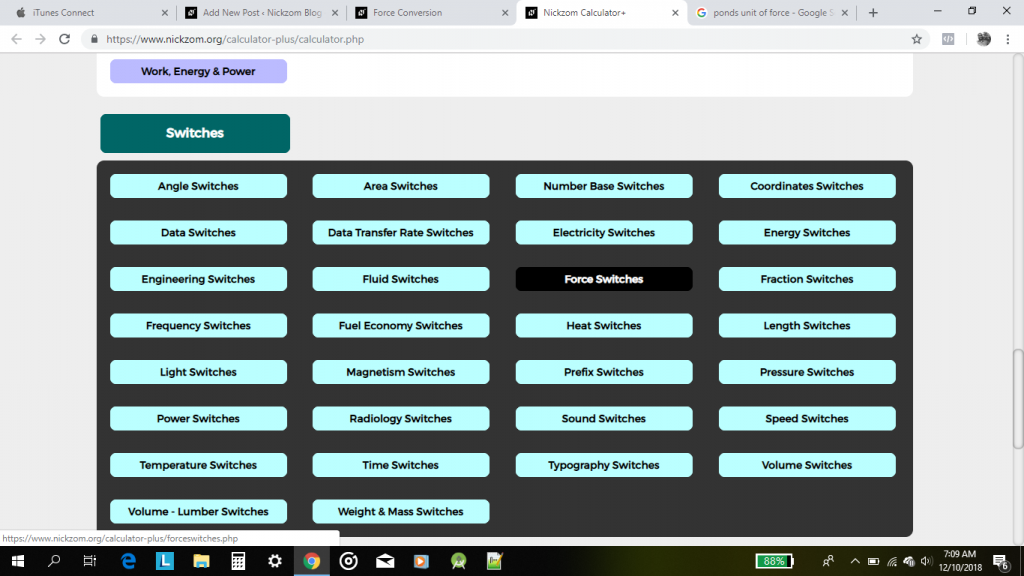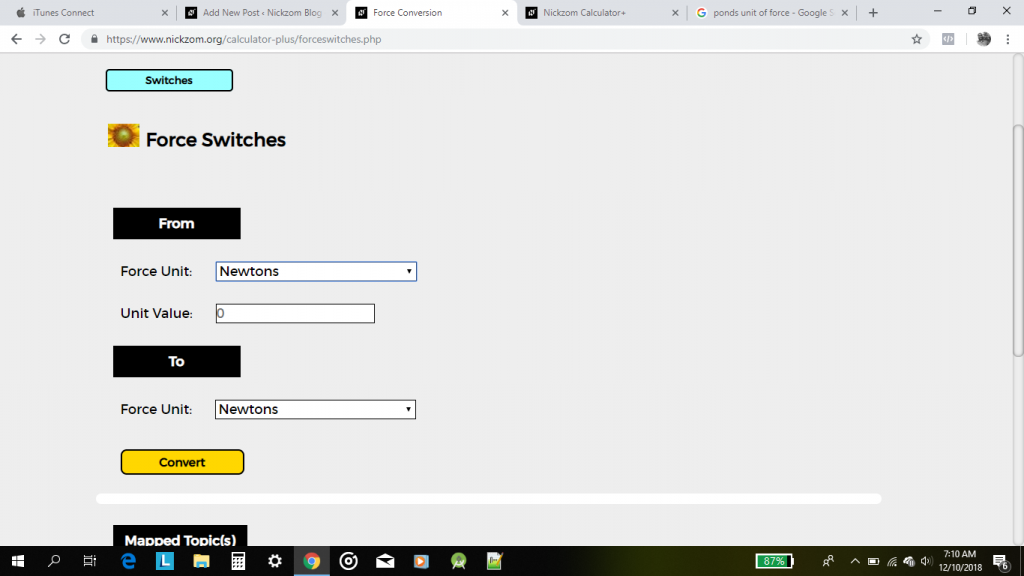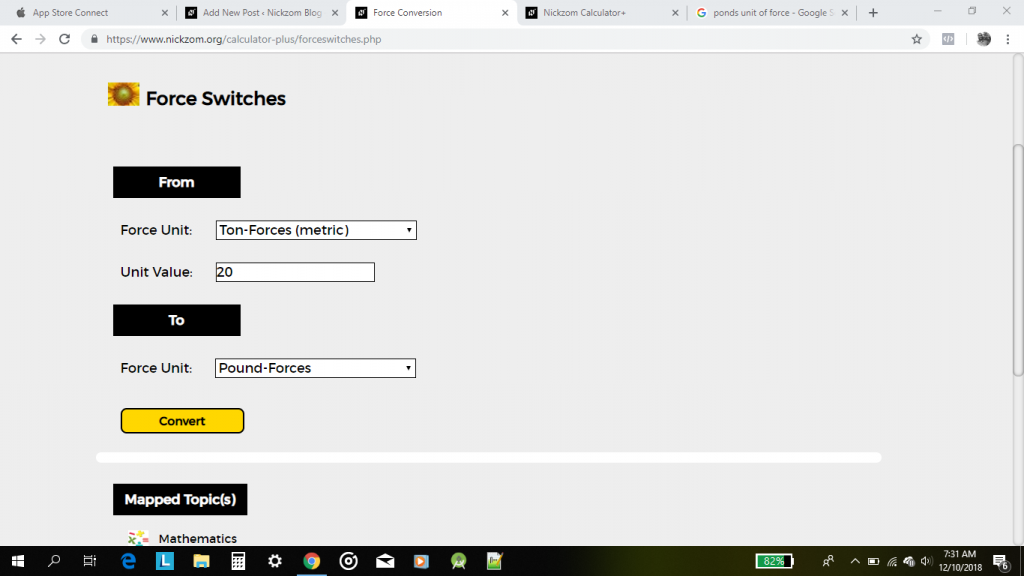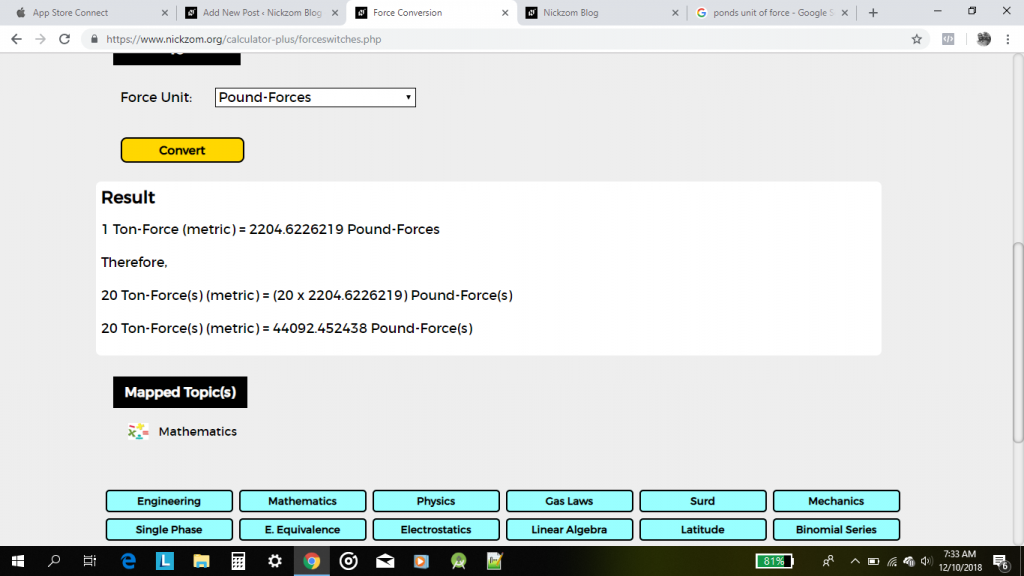# How to Convert From One Unit of Force to Another Unit of Force

Nickzom Calculator is a calculator encyclopedia tool that converts units of force. There is a large number of force units and the units that can be converted using Nickzom Calculator are:

• Newtons
• Kilonewtons
• Gram-Forces
• Kilogram-Forces
• Ton-Forces (metric)
• Exanewtons
• Petanewtons
• Teranewtons
• Giganewtons
• Meganewtons
• Hectonewtons
• Dekanewtons
• Decinewtons
• Centinewtons
• Millinewtons
• Micronewtons
• Nanonewtons
• Piconewtons
• Femtonewtons
• Attonewtons
• Dynes
• Joules/meter
• Joules/centimeter
• Ton-Forces (Short)
• Ton-Forces (Long)
• Kip-Forces
• Kilopound-Forces
• Pound-Forces
• Ounces-Forces
• Poiundals
• Pound Feet/Sq. Second
• Ponds
• Kiloponds

Let’s convert 20 Ton-Forces (metric) to Pound-Forces.

First and Foremost, proceed to the Calculator Map, click on Force Switches under Switches section.On clicking, displays the interface for you to enter your values.Now to perform the example above we have to select the appropriate units in From and To and enter the accurate unit value.

From the example given, the From Force Unit is Ton-Forces (metric), the Unit Value is 20 and the To Force Unit is Pound-Forces.Finally, click on the Convert buttonHere goes the result of the conversion as stated in the screenshot above.

You can access Nickzom Calculator (The Calculator Encyclopedia) via any of these channels:

## 2 thoughts on “How to Convert From One Unit of Force to Another Unit of Force”

1.www.iamsport.org says:

Great article, exactly what I needed.